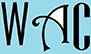## Algebra Question for Title 1 Schools: Solve for X Question?

5) Solve for x: 2x/3 = 8 + 4x

you have to make “x” subject of formula by moving all the “x” to one side. When you go to the other side, addition becomes subtraction, multiplication becomes division, and vice versa.

First we deal with the 3

2x/3 = 8 + 4x

2x = 3(8 + 4x) – 3 was a divisor at the other end

2x = 24 + 12x

2x – 12 x = 24 (12x was addition at the other end)

-10x = 24

x = 24/-10 (-10 was multiplication at the other end)

x = -2.4